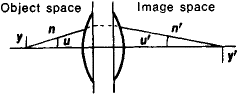# Abbe Sine Condition

Also found in: Medical, Wikipedia.
The following article is from The Great Soviet Encyclopedia (1979). It might be outdated or ideologically biased.

## Abbe Sine Condition

a condition that must be fulfilled if an optical system corrected for spherical aberration is to produce an undistorted, or nonaberrational, image y′ of a small linear element y that is located on the optical axis of the system and is perpendicular to this axis (see Figure 1). The condition is expressed by the equation sin u/sin u′ = βn′/n. Here, u and u′ are the angles formed with the optical axis by a ray passingFigure 1

through an object point and an image point that are located on the axis, n and n′ are the refractive indexes of the media on the two sides of the optical system, and β = y/y is the linear magnification of the system.